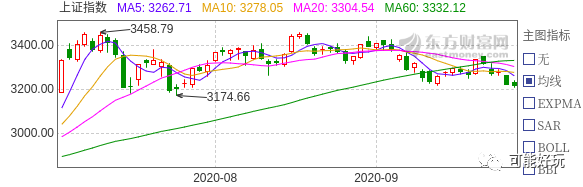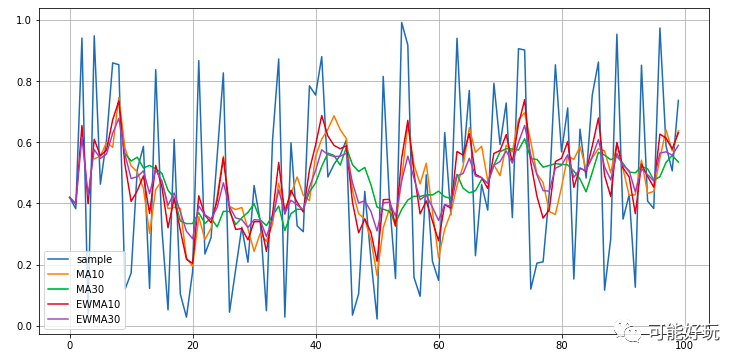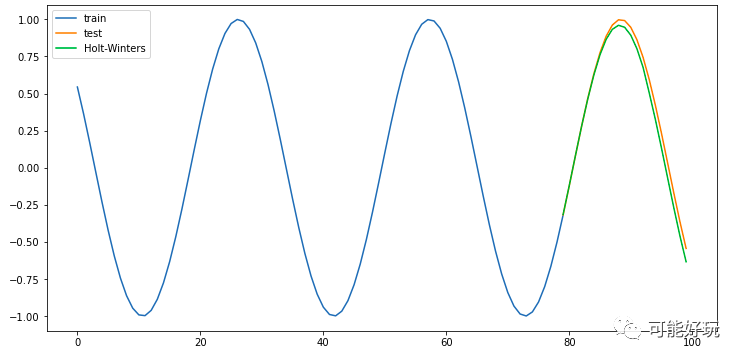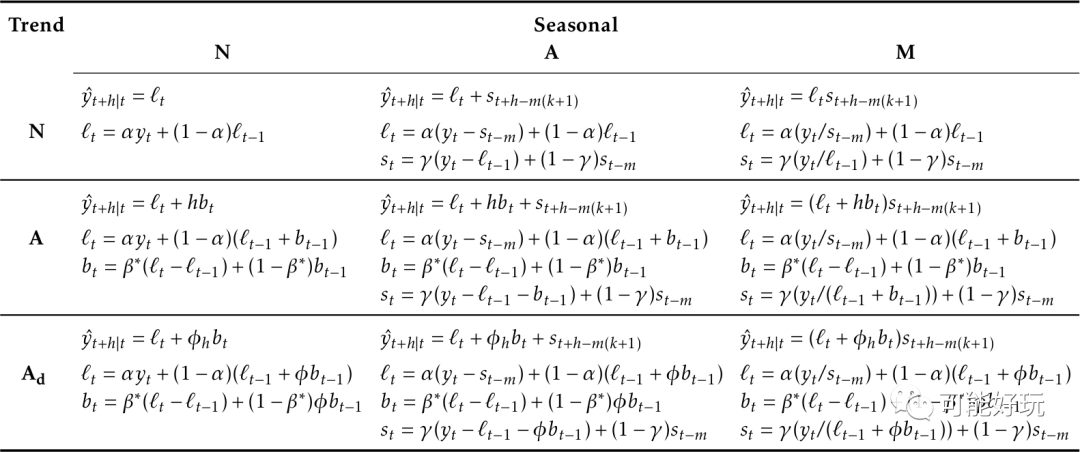# 移动平均

## 移动平均线image-20200925174725577

「滞后性」

「趋势性」

## 指数加权移动平均

ewma与MA的区别是，MA通常是以某个大小的时间窗口去计算，而ewma是计算所有样本的，但是会强调当前样本的重要性，逐渐淡化历史样本的重要程度。

「公式：」

## python代码

import pandas as pdimport numpy as npimport matplotlib.pyplot as plt#生成均匀分布数据data = pd.DataFrame()data['sample'] = np.random.normal(size=100)#简单移动平均data['MA5'] = data['sample'].rolling(window=5).mean()data['MA10'] = data['sample'].rolling(window=10).mean()#指数加权移动平均data['EWMA5'] = data['sample'].ewm(span=5).mean()data['EWMA10'] = data['sample'].ewm(span=10).mean()data[['sample','MA5','MA10','EWMA5','EWMA10']].plot(subplots=False, figsize=(12,6), grid=True)image-20200925181921625

# 指数平滑

，是线性平滑模型参数

m为预测超前期数

## 三次指数平滑

「趋势调整」

1、 利用前面介绍的方法计算第t期的简单指数平滑预测；

2、 计算趋势。其公式为：

=第t期经过平滑的趋势；

=第t期上期经过平滑的趋势；

b=选择的趋势平滑系数；

=对第t期简单指数平滑预测；

=对第t期上期简单指数平滑预测。

3、计算趋势调整后的指数平滑预测值.计算公式为：

「的确定」

α值是根据时间序列的变化特性来选取的。若时间序列的波动不大，比较平稳，则α应取小一些，如0.1 ～ 0.3 ；若时间序列具有迅速且明显的变动倾向， 则α应取大一些，如0.6 ～ 0.9。实质上，α是一个经验数据，通过多个值进行试算比较而定，哪个α值引起的预测误差小，就采用哪个。

ps:发现网络上都是把三次指数平滑法和holt-winters当同一个东西，但是有的三次指数平滑公式又不一样0-0，稍微查了一下资料，上面的三次指数平滑法是布朗指数平滑。下面的又是另一种。

## Holt-Winters

Holt-Winters季节性方法包括预测方程和三个平滑方程-一个水平(level)的，一个趋势方程，一个用于季节性成分 ，具有相应的平滑参数 ，，。我们用表示季节性的频率，即一年中的季节数。例如，对于季度数据L=4，对于每年的中的月则是

「加法模型」

「乘法模型」

「衰减模型」

### python代码

import pandas as pdimport numpy as npimport matplotlib.pyplot as pltimport seaborn as sns#导入库from statsmodels.tsa.holtwinters import ExponentialSmoothing#生成sin数据data = pd.DataFrame()a = np.linspace(-10, 10, 100)b = np.sin(a)data['sin']=btrain,test = data.iloc[:80,0],data.iloc[79:,0]model = ExponentialSmoothing(train,seasonal='add',seasonal_periods=31).fit()pred = model.predict(start=test.index,end=test.index[-1])fig=plt.figure(figsize=(12,6))plt.plot(train.index,train,label='train')plt.plot(test.index,test,label='test')plt.plot(pred.index,pred,label='Holt-Winters')plt.legend(loc='best')plt.show()image-20200925184544024

「seasonal_periods」参数的作用就是季节波动，这里设置的是31，几乎完美拟合曲线

## 指数平滑法的组合分类img

# Reference

1. https://wiki.mbalib.com/wiki/%E6%8C%87%E6%95%B0%E5%B9%B3%E6%BB%91%E6%B3%95#.EF.BC.88.E4.B8.89.EF.BC.89_.E4.B8.89.E6.AC.A1.E6.8C.87.E6.95.B0.E5.B9.B3.E6.BB.91.E9.A2.84.E6.B5.8B
2. https://en.wikipedia.org/wiki/Exponential_smoothing#Triple_exponential_smoothing_(Holt_Winters)
3. https://otexts.com/fpp2/taxonomy.html

11-285710-12776
01-061万+
12-022
12-04658
11-2312
12-04396
12-15367
02-112506
11-2845
11-2317
11-2316
07-18460
11-248
09-23176
12-23127
10-09417
11-2548
11-07540
©️2020 CSDN 皮肤主题: 深蓝海洋 设计师:CSDN官方博客